Vectors and conics

This free course is available to start right now. Review the full course description and key learning outcomes and create an account and enrol if you want a free statement of participation.

Free course

1.9 Distance between points in three-dimensional Euclidean space

You saw in Section 1.5 that the distance between two points (x1, y1) and (x2, y2) in the plane is given by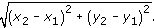We can establish a similar formula for the distance between two points in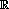3, as follows.

Let P (x1, y1, z1) and Q(x2, y2, z2) be two points in3. Let M be the foot of the perpendicular from Q to the plane through P that is parallel to the (x, y)-plane; then M has coordinates (x2, y2, z1). Next, let N be the point in this plane with coordinates (x1, y2, z1).

Then the triangles PQM and PMN are both right-angled triangles, with right angles at M and N, respectively, as shown below.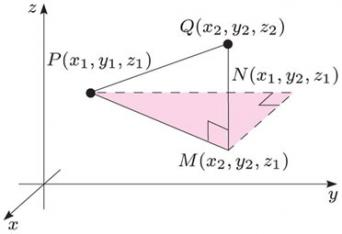The length of PN is |y2y1|, the length of NM is |x2x1|, and the length of MQ is |z2z1|. It follows from Pythagoras' Theorem that

PM2 = NM2 + PN2,

so

PM2 = (x2x1)2 + (y2y1)2.

Note: This part of the discussion is similar to the derivation of the Distance Formula in2 (see Section 1.5).

Using Pythagoras' Theorem again, we obtain

PQ2 = PM2 + MQ2

so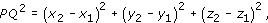that is,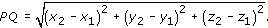Distance Formula in three-dimensional Euclidean space

The distance between two points (x1, y1, z1) and (x2, y2, z2) in3 is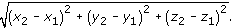For example, it follows from this formula that the distance between the points (1, 2, 3) and (4, −2, 15) is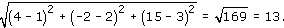Example 11

For each of the following pairs of points in3, find the distance between them.

• (a) (1, 1, 1) and (4, 1, −3).

• (b) (1, 2, 3) and (3, 0, 3).

We use the formula for the distance between two points in3 This gives the following distances.

•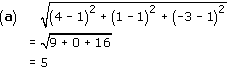•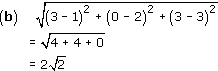M208_1